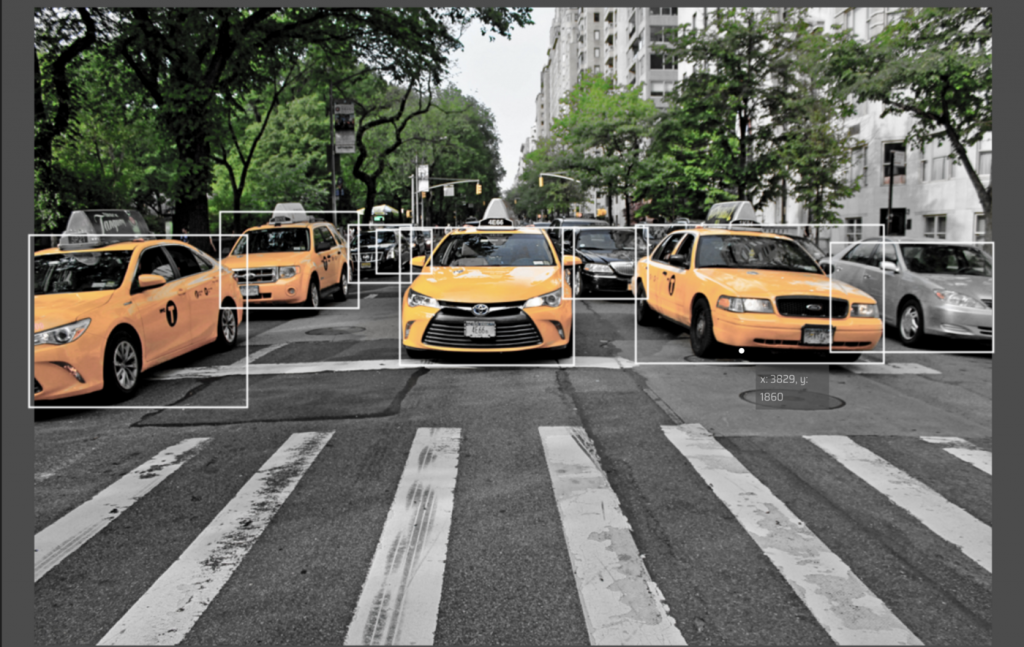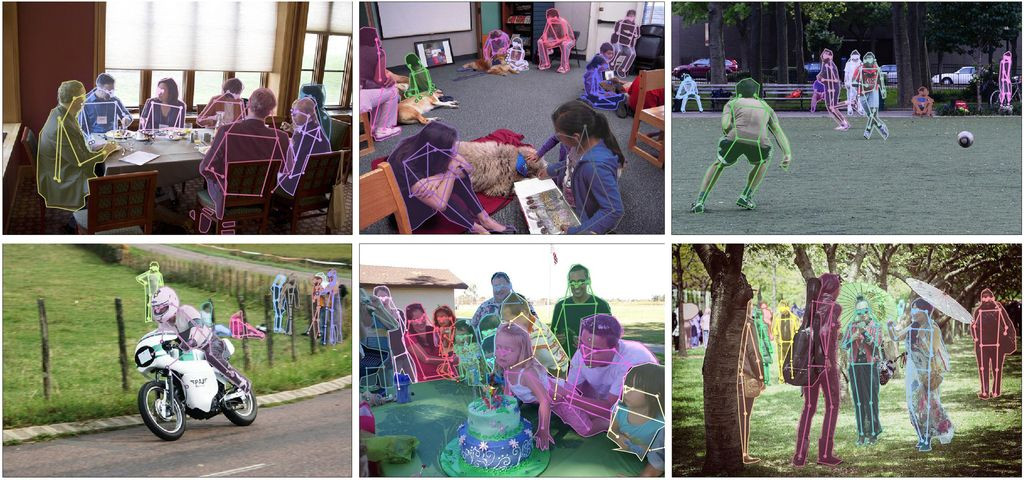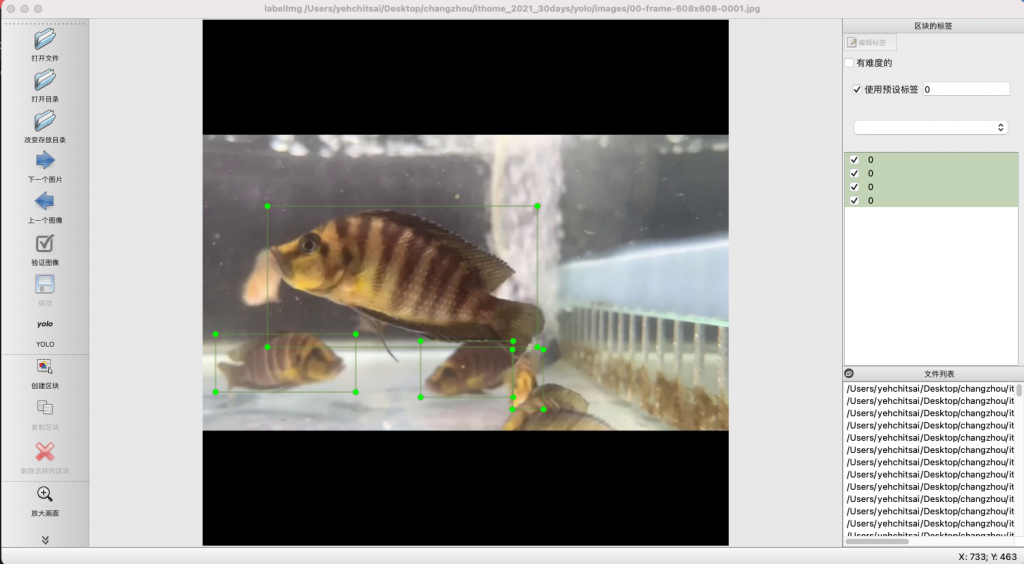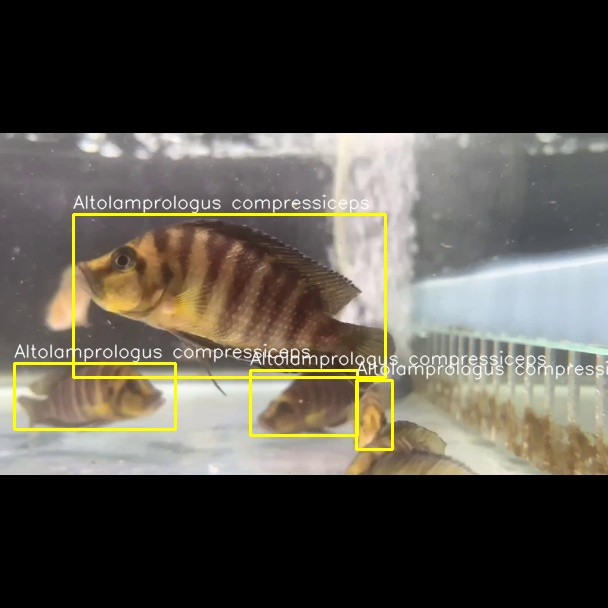DAY 12
0
AI & Data

# 標籤圖片的方法與實作 － Day 12

1. 圖片標註的類型 (Image Annotation Types)：可以是邊界框 (Bounding boxes)，多邊形分割 (Polygonal Segmentation)，語義分割 (Semantic Segmentation)，3D 長方體 (3D cuboids)，關鍵點和界標 (Key-Point and Landmark)，直線和樣條 (Lines and Splines)。下圖顯示邊界框與關鍵點和界標的圖型。使用邊界框來標註物件是很常見的標註方式，將目標物件用矩型標註出來，YOLOV3也是使用這種方式。1. 圖片標註的格式 (Image Annotation Formats)：可以分成 COCO，Pascal VOC 與 YOLO 三種不同的格式。簡單來區分是 COCO 使用的是 JSON 的格式；Pascal VOC 使用 XML 格式；而 YOLO 則是一般文字格式，可以說是類似 CSV 格式，只是都是以空白來進行不同欄位。以下分別是他們的簡單樣板。

COCO format

``````annotation{
"id" : int,
"image_id": int,
"category_id": int,
"segmentation": RLE or [polygon],
"area": float,
"bbox": [x,y,width,height],
"iscrowd": 0 or 1,
}
categories[{
"id": int,
"name": str,
"supercategory": str,
}]
``````

Pascal VOC

``````<annotation>
<folder>Train</folder>
<filename>01.png</filename>
<path>/path/Train/01.png</path>
<source>
<database>Unknown</database>
</source>
<size>
<width>224</width>
<height>224</height>
<depth>3</depth>
</size>
<segmented>0</segmented>
<object>
<name>36</name>
<pose>Frontal</pose>
<truncated>0</truncated>
<difficult>0</difficult>
<occluded>0</occluded>
<bndbox>
<xmin>90</xmin>
<xmax>190</xmax>
<ymin>54</ymin>
<ymax>70</ymax>
</bndbox>
</object>
</annotation>
``````

``````<object-class> <x_iw_ratio> <y_ih_ratio> <tw_iw_ratio> <th_ih_ratio>
``````

``````# Pascal VOC
ymin, xmin, ymax, xmax, image_w, image_h = PascalVOC

# 將 Pascal VOC 中的資料轉換成 YOLO 格式
(x_iw_ratio, y_ih_ratio) = ( ( (xmin + xmax) * 0.5 ) / image_w, ((ymin + ymax) * 0.5 ) / image_h)
tw_iw_ratio = (xmax - xmin) * 1. / image_w
th_ih_ratio = (ymax - ymin) * 1. / image_h
``````
1. 標籤工具：標籤工作可以簡單的分成半自動跟手動，半自動的標籤工具有：Anno-Mage，顯示出來的自動標示功能還不錯，只是已經 1,2 年沒有繼續維護了；而大多數是用手動工具 labelImg

00-frame-0001.txt

``````0 0.962963 0.447917 0.414815 0.137500
0 0.263889 0.422917 0.416667 0.122917
``````./utils/yoloDrawLabel.py

``````import cv2

# 設定輸入與輸出
inputLabelPath = '../labels/00-frame-0001.txt'
inputImgPath = '../images/00-frame-608x608-0001.jpg'
outputImgPath = '../labels/00-frame-608x608-0001.jpg'
# 圖片大小固定為 608＊608
img_w= img_h = 608
# 載入圖片

with open(inputLabelPath) as f:
for line in f:
line = line.strip() # 刪除多餘的空白
data = line.split() # 將 YOLO 一列的內容轉成一維陣列
# 將 YOLO 格式轉換為邊界框的左上角和右下角座標
bbox_width = float(data) * img_w
bbox_height = float(data) * img_h
center_x = float(data) * img_w
center_y = float(data) * img_h
min_x, min_y = center_x - (bbox_width / 2), center_y - (bbox_height / 2)
max_x, max_y = center_x + (bbox_width / 2), center_y + (bbox_height / 2)
print(min_x,min_y,max_x,max_y)
# 在圖片上寫上物件名稱
cv2.putText(cv2image, 'Altolamprologus compressiceps', (int(min_x), int(min_y-6)), cv2.FONT_HERSHEY_SIMPLEX, 0.6, (255, 255, 255), 1, cv2.LINE_AA)
# 畫出邊界框
cv2.rectangle(cv2image, (int(min_x),int(min_y)), (int(max_x),int(max_y)), (0,255,255), 2)
# 將檔案另存新圖片檔
cv2.imwrite(r'{}'.format(outputImgPath), cv2image)
f.close()
``````